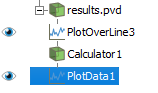# Plotting univariate function to compare against simulation results

I was wondering today how to plot a known shear-stress profile against computed results. Came up with a solution, thought I’d share.

Let’s say I have the following data that I’ve created via PlotOverLine filters from a simulation solution:

And I want to create the actual function f(x) = \left(\frac{3}{2}\right)\left(\frac{-1e^{-6}}{1}\right)\left( 1 -\frac{x^2}{0.5^2}\right) and plot it on the same chart.

It seems like the easiest way is to:

1. Apply a Calculator filter to the PlotOverLine filter
• Use the arc_length variable as the independent variable, this variable is generated by the PlotOverLine filter
2. Apply a PlotData filter to the Calculator
• The Series Parameters data array to plot has the same name as specified in the Calculator filter’s Result Array Name field.

Note that since arc_length is in the range [0, 1] and I know that I need x = [-0.5, 0.5] I had to shift my function in the Calculator filter:

( 1.5 ) * ( -1e-6 ) * ( 1 - ( ( ( arc_length - 0.5 )^2 ) / ( 0.5^2 ) ) )1 Like